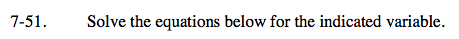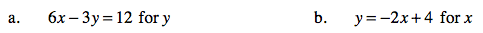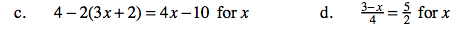Home > CAAC > Chapter 7 > Lesson 7.1.5 > Problem7-51

7-51.
1. Solve the equations below for the indicated variable. Homework Help ✎

1. 6x − 3y = 12 for y

2. y = −2x + 4 for x

3. 4 − 2(3x + 2) = 4x − 10 for x

4.=for xSolve for the variable by isolating it on one side.

−3y = 12 − 6x

Now divide both sides by −3.

Follow the steps outlined in part (a).First distribute to remove the parenthesis, then combine like terms. To finish, follow steps in part (a).

x = 1

Multiply by a common denominator to remove the fractions. Then follow the steps in part (a).